Next: Divisive clustering Up: Hierarchical clustering Previous: Centroid clustering   Contents   Index

# Optimality of HAC

To state the optimality conditions of hierarchical clustering precisely, we first define the combination similarity COMB-SIM of a clustering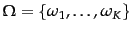as the smallest combination similarity of any of its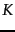clusters: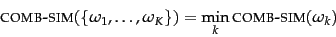(210)

Recall that the combination similarity of a clusterthat was created as the merge of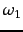and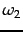is the similarity ofand(page 17.1 ).

We then defineto be optimal if all clusterings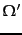with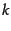clusters,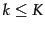, have lower combination similarities: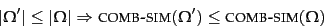(211)

Figure 17.12 shows that centroid clustering is not optimal. The clustering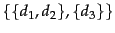(for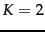) has combination similarity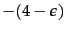and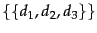(for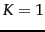) has combination similarity -3.46. So the clusteringproduced in the first merge is not optimal since there is a clustering with fewer clusters () that has higher combination similarity. Centroid clustering is not optimal because inversions can occur.

The above definition of optimality would be of limited use if it was only applicable to a clustering together with its merge history. However, we can show (Exercise 17.5 ) that for the three non-inversion algorithms can be read off from the cluster without knowing its history. These direct definitions of combination similarity are as follows.

The combination similarity of a clusteris the smallest similarity of any bipartition of the cluster, where the similarity of a bipartition is the largest similarity between any two documents from the two parts: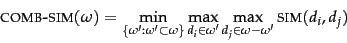(212)

where each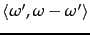is a bipartition of.
The combination similarity of a clusteris the smallest similarity of any two points in: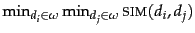.
GAAC
The combination similarity of a clusteris the average of all pairwise similarities in(where self-similarities are not included in the average): Equation 205.
If we use these definitions of combination similarity, then optimality is a property of a set of clusters and not of a process that produces a set of clusters.

We can now prove the optimality of single-link clustering by induction over the number of clusters. We will give a proof for the case where no two pairs of documents have the same similarity, but it can easily be extended to the case with ties.

The inductive basis of the proof is that a clustering with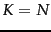clusters has combination similarity 1.0, which is the largest value possible. The induction hypothesis is that a single-link clustering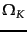withclusters is optimal: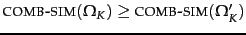for all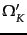. Assume for contradiction that the clustering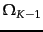we obtain by merging the two most similar clusters inis not optimal and that instead a different sequence of merges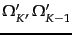leads to the optimal clustering with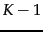clusters. We can write the assumption that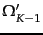is optimal and thatis not as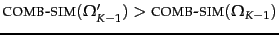.

Case 1: The two documents linked by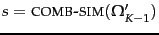are in the same cluster in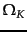. They can only be in the same cluster if a merge with similarity smaller than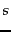has occurred in the merge sequence producing. This implies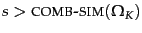. Thus,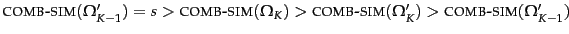. Contradiction.

Case 2: The two documents linked byare not in the same cluster in. But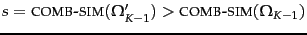, so the single-link merging rule should have merged these two clusters when processing. Contradiction.

Thus,is optimal.

In contrast to single-link clustering, complete-link clustering and GAAC are not optimal as this example shows: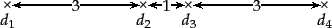Both algorithms merge the two points with distance 1 (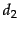and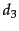) first and thus cannot find the two-cluster clustering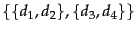. Butis optimal on the optimality criteria of complete-link clustering and GAAC.

However, the merge criteria of complete-link clustering and GAAC approximate the desideratum of approximate sphericity better than the merge criterion of single-link clustering. In many applications, we want spherical clusters. Thus, even though single-link clustering may seem preferable at first because of its optimality, it is optimal with respect to the wrong criterion in many document clustering applications.

Table 17.1: Comparison of HAC algorithms.
 method combination similarity time compl. optimal? comment single-link max inter-similarity of any 2 docs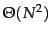yes chaining effect complete-link min inter-similarity of any 2 docs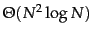no sensitive to outliers group-average average of all simsno best choice for most applications centroid average inter-similarityno inversions can occur

Table 17.1 summarizes the properties of the four HAC algorithms introduced in this chapter. We recommend GAAC for document clustering because it is generally the method that produces the clustering with the best properties for applications. It does not suffer from chaining, from sensitivity to outliers and from inversions.

There are two exceptions to this recommendation. First, for non-vector representations, GAAC is not applicable and clustering should typically be performed with the complete-link method.

Second, in some applications the purpose of clustering is not to create a complete hierarchy or exhaustive partition of the entire document set. For instance, first story detection or novelty detection is the task of detecting the first occurrence of an event in a stream of news stories. One approach to this task is to find a tight cluster within the documents that were sent across the wire in a short period of time and are dissimilar from all previous documents. For example, the documents sent over the wire in the minutes after the World Trade Center attack on September 11, 2001 form such a cluster. Variations of single-link clustering can do well on this task since it is the structure of small parts of the vector space - and not global structure - that is important in this case.

Similarly, we will describe an approach to duplicate detection on the web in Section 19.6 (page 19.6 ) where single-link clustering is used in the guise of the union-find algorithm . Again, the decision whether a group of documents are duplicates of each other is not influenced by documents that are located far away and single-link clustering is a good choice for duplicate detection.

Exercises.

• Show the equivalence of the two definitions of combination similarity: the process definition on page 17.1 and the static definition on page 17.5 .Next: Divisive clustering Up: Hierarchical clustering Previous: Centroid clustering   Contents   Index